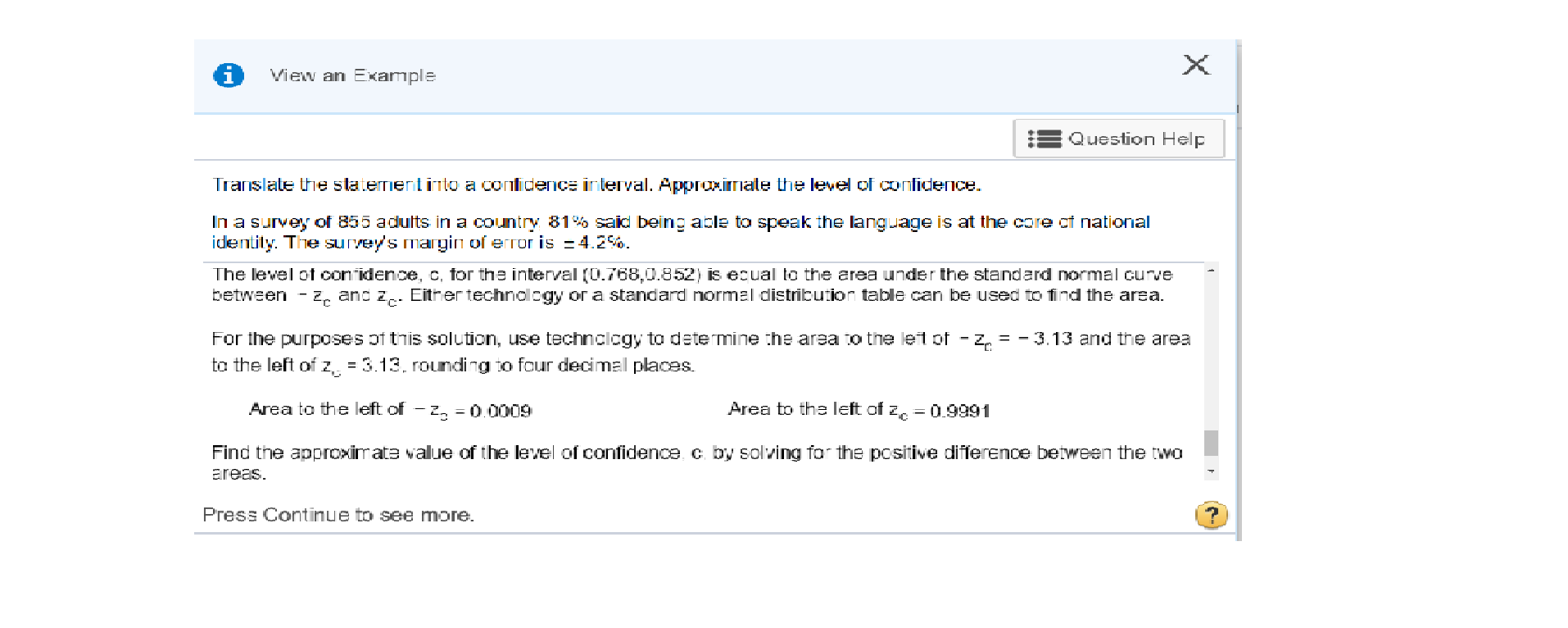# View an ExampleQuestion HelpTranslate the staternentinto a conlidence interval. ApproximIate the level of conlideceIn a survey of 855 adults in a country 81 said being able to speak the language is at the core ct nationalidentily. The survey's margin of error is 4.2%The level of conidence, C, for the interval (0.768,0.852) is ecual to the area under the standard normal curvebetween - Z, and z. Either technolcgy or a standard normal distribution table can be used to find the area.For the purposes of this solution, use technclcgy to determine the area to the let of- z = -3.13 and the areato the left of z, 3.13. rouding to four decimal placesArea to the left of zArea to the left of - z= 0.00090.9991Find the approximate value of the level of confidence c by solving for the pcsitive difference between the twoareasPress Continue to see more.

Question
6 views

How can you find the left area of  zc= -3.13and -zc values using a ti-83 calculatorhelp_outlineImage TranscriptioncloseView an Example Question Help Translate the staternentinto a conlidence interval. ApproximIate the level of conlidece In a survey of 855 adults in a country 81 said being able to speak the language is at the core ct national identily. The survey's margin of error is 4.2% The level of conidence, C, for the interval (0.768,0.852) is ecual to the area under the standard normal curve between - Z, and z. Either technolcgy or a standard normal distribution table can be used to find the area. For the purposes of this solution, use technclcgy to determine the area to the let of- z = -3.13 and the area to the left of z, 3.13. rouding to four decimal places Area to the left of z Area to the left of - z = 0.0009 0.9991 Find the approximate value of the level of confidence c by solving for the pcsitive difference between the two areas Press Continue to see more. fullscreen
check_circle

Step 1

Follow the provided steps of Ti-83 calculator to find out the area to the left of -3.13

1. Press 2nd then DISTR
2. Select normalcdf(
3. Press (-) which is to the left of Enter key > Type 1 > Press 2nd , EE (on comma key) and write 99.
4. Type -3.13 and close the right bracket.
5. Press Enter.
Step 2

The obtained output i...

### Want to see the full answer?

See Solution

#### Want to see this answer and more?

Solutions are written by subject experts who are available 24/7. Questions are typically answered within 1 hour.*

See Solution
*Response times may vary by subject and question.
Tagged in

### Other